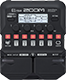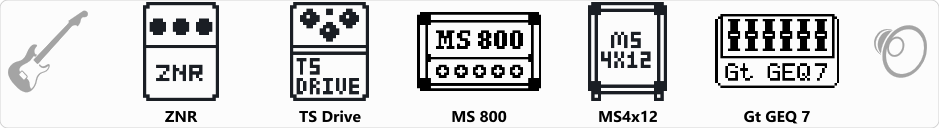# JCM800 Distortion

Discussion in 'Zoom G1/G1X Four' started by lastPanda, Jan 30, 2020.

1. JCM800 DistortionDevice: Zoom G1 Four
Firmware: 2.00

Name on device: JCM800
Optimized for: Phones/Speaker

Effects chain:JCM800 Distortion

Effect: "ZNR" (Dynamics), active - "yes"
"Detect" = GTRIN
"Depth" = 50
"Threshold" = 40
"Decay" = 0

Effect: "TS Drive" (Overdrive / Distortion), active - "yes"
"Gain" = 0
"Boost" = On
"Tone" = 50
"Volume" = 70

Effect: "MS 800" (Amp simulator), active - "yes"
"Input" = HI
"Bass" = 50
"Middle" = 50
"Treble" = 50
"Presence" = 30
"Gain" = 70
"Volume" = 65

Effect: "MS4x12" (Cabinet), active - "yes"
"MIC" = ON
"D57: D421" = 0
"Hi" = 50
"Lo" = 50

Effect: "Gt GEQ 7" (Filter), active - "yes"
"100" = 1.0
"200" = 0.0
"400" = -3.0
"800" = 0.0
"1.6k" = 0.0
"3.2k" = 0.0
"6.4k" = 0.0
"VOL" = 80

Patch Volume: 82